# Test Data

## Overview of Tests

The data bank consists of data for two main cases. The first case is on-cam i.e. the top-point (T) on the propeller curve (single runner blade angle curve). The second case is off-cam i.e. the right-leg (R) on the propeller curve. Both are conducted at 60 % load, which is close to the best efficiency for the system, and at the same test head (H = 4.5 m).

The measurements were carried out during a period of five years. The model broke during this period and it was not possible to exactly repeat the same conditions after the accident. Therefore the data has been divided into before (r) and after (n), e.g. T(r) in the text (or Tr in file names) refers to the early Case T measurements. A more thorough discussion, that describes the different operational modes, can be found in Andersson (2003a). The (r)-measurements were used as initial conditions for both Turbine 99-workshops, while (n)-measurements are more complete with data at outlet section II and III.

The exact settings of the runner speed, N, and the resulting flow, Q, (i.e. PDPs) and the corresponding unit speeds, N11 and Q11 (i.e. GNDPs), are shown in Table EXP-A. In Figure 2.1 one can see an overview of the relation between the operational modes.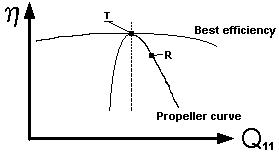Figure 2.1: Sketch of the propeller curve and location of the test cases.

The flow of the draft tube has been studied with visualisations to get an overview of the main flow regimes. LDV has been used at a number of cross sections for velocity measurements. Wall pressure measurements has been performed to evaluate the performance of the draft tube. Figure 1.3 and 1.4 gives a description of the geometric location of each type of measurement. The available data has been listed in Table EXP-B.

In Andersson and Karlsson (1999) and Andersson (2003 a,b) a presentation of the methods used and a thorough discussion of the quality of the experimental data can be found.

Table EXP-A Summary Description of All Test Cases
Name GNDPs PDPs MPs
N11

(√m/60s)

Q11

(√m/s)

ReH

(10-6)

(m)

Flow rate

(m3/s)

Runner speed

(rpm)

Detailed Data DOAPs
EXP 1a

(T(r) - case)

140.4 1.00 4.1 4.5 0.528 595 Cp, U, v, k α, β, S
EXP 1b

(T(n) - case)

140.4 0.98 4.1 4.5 0.522 595 Cp, U, v, k Cpr wall Cpr average

ζ, α, β, S

EXP 2a

(R(r) - case)

140.4 1.03 4.1 4.5 0.548 595 Cp, U, v, k α, β, S
EXP 2b

(R(n) - case)

140.4 1.02 4.1 4.5 0.542 595 Cp, U, v, k Cpr wall Cpr average

ζ, α, β, S

## Test case DATA

### Description of Experiment

Case T and R are a collection of all experimental data, collected at each operational mode. The setting of GNDPs and PDPs can be found in Table EXP-A for each case. In section 1.4 a description of the geometric location of each type of measurement is given.

This paragraph is followed by a brief description of the available boundary conditions, a presentation of typical measurement errors at each section. After that follows a listing of available data files, first for Case T and second for Case R.

### Boundary Data

The velocity measurements at section Ia serve as inlet conditions for the draft tube. These include the axial and the tangential mean and RMS velocity components. However, the radial velocity component is missing. More details can be found Gebart, Gustavsson and Karlsson (2000).

The model walls are painted steel surfaces and the surface roughness is estimated to be in the order of 10 μm, therefore the walls can be considered to be hydraulically smooth with the typical velocities in the experiment. Between model parts there can be discontinuities and weld seams that can cause local losses.

In Andersson (2003c) additional information e.g. about periodic behaviour at the inlet can be found. Also see section 3.2.3. regarding assumptions and settings used and recommended for CFD calculations.

### Measurement Errors

In Andersson and Karlsson (1999) a thorough discussion of the quality of the experimental data can be found. Typical results from the evaluation can be seen in Table 1 and 2 (for case T). There is no major difference between case T and R. These are averaged values. The individual error estimation for each raw data value can be found in the supplied raw data files.

 C.s. Ia C.s. Ib C.s. III Random error ± 2.0 % / ± 3.5 % ± 2.0 % / ± 3.5 % ± 3.0 % / ± 5.0 % Velocity bias (axial component) < +0.9 % < + 1.4 % < + 3.6 % Probe volume location ± 1 % / ± 1-2 % ± 0.5 % / ± 0.5-5% ± 0.25 % / ± 0.25 % Leakage - 2 % - - Symmetry ± 1 % / ± 1.25 % ± 2 % / ± 2.5 % - Total error axial tangential x1 < ε < x2 - 4.1 % +1.3 % - 5.2 % + 3.1 % x1 < ε < x2 - 2.0 % + 4.3 % >-5.9 % <+7.9 % x1 < ε < x2 - 1.5 % + 6.6 % - 3.5 % + 8.6 %

 C.s. Ia * C.s. Ib C.s. III Umean [m/s] 3.54 2.44 1.01 Vmean [m/s] 0.82 0.69 0.08 Q_int/Q [ ] 0.97 1.03 1.00
* mean values for the two measured cross sections

### Measured Data

The raw data files (*.dat, see below) are stored in ASCII-format. If nothing else is specified the format of the files used, is:

position(x, y and z-coordinates) mean values rms values error margins

Velocity data is normalised with the mean value of the local average velocity (Q/Area of cross section) and pressure data is normalised with the dynamic pressure at the inlet and the pressure reference level is set to one at section IVb. The data has not been interpolated or otherwise manipulated if nothing else is stated.

In Andersson (1999, 2003a, 2003b) most of the data is presented and the main flow features discussed. (All contributions to Turbine 99-Workshop 2 are collected in the proceedings, Engström et al (2003)).

Exp 1a and 1b: Case T

Derived data:

DOAPs calculated at available cross sections for case T(r): TrDOAP.dat

DOAPs calculated at available cross sections for case T(n): TnDOAP.dat

#### Visualisations

A number of pictures that describes the flow for Case T. The visualisations have focused on the streamlines close to the wall.

The pictures are stored in jpeg-format.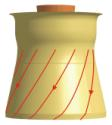Figure 2.2: Streamlines close to the wall at the inlet cone.

 A. Left view B. Top view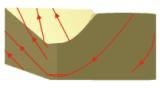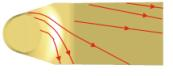C. Right view D. Downstream view (c.s. III)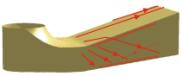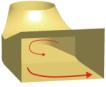Figure 2.3: Streamlines close to the surface at the elbow and outlet diffuser.

Velocity and pressure measurements:

Section Ia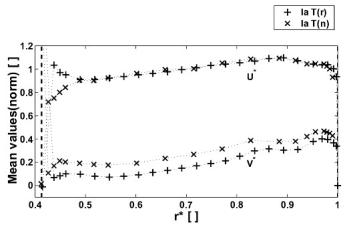Figure 2.4: The normalised mean velocity components at profile Ia(1).

Normalised axial and tangential velocity measurements at cross section Ia,
along profile 1 (-10°), for Case T(r). (See Figure 1.5).

Normalised axial and tangential velocity measurements at cross section Ia,
along profile 2 (180°), for Case T(r). (See Figure 1.5).

Normalised axial and tangential velocity and pressure measurements
at cross section Ia, along profile 1 (-10°), for Case T(n). (See Figure 1.5).

Normalised static and dynamic pressure measurements at cross section Ia,
along profile 3 ( -80°), for Case T(n). (See Figure 1.5).

Normalised wall pressure measurements at cross section Ia,
for Case T(n). (See Figure 1.5).

Section Ib

Normalised axial and tangential velocity and pressure measurements
at cross section Ib, along profile 1 (-80°), for Case T(r). (See Figure 1.5).

Normalised axial and tangential velocity and pressure measurements
at cross section Ib, along profile 1 (-80°), for Case T(n). (See Figure 1.5).

Section II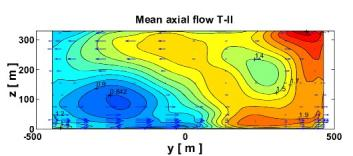Figure 2.5: The axial (contours) and horizontal (vectors) velocity components at c.s. II (down stream view).

Normalised axial and tangential velocity and pressure measurements
at cross section II for Case T(n). (See Figure 1.4).

Section III

Normalised axial and tangential velocity and pressure measurements
at cross section III, for Case T(n). (See Figure 1.4).

Section IVb

Normalised wall pressure measurements at cross section IVb, for Case T(r).
(See Figure 1.4).

Centrelines

Normalised wall pressure measurements along the upper centre line,
for Case T(n). (See Figure 1.7).

Normalised wall pressure measurements along the lower centre line,
for Case T(n). (See Figure 1.7).

Exp 2a and 2b Case R

Derived data:

DOAPs calculated at available cross sections for case R(r).

DOAPs calculated at available cross sections for case R(n).

Visualisations:

A number of pictures that describe the flow for Case R. The visualisations have focused on the streamlines close to the wall.

The pictures are stored in jpeg-format.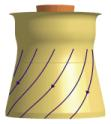Figure 2.6: Streamlines close to the wall at the inlet cone.

 A. Left view B. Top view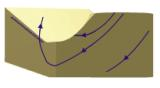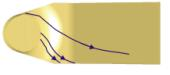C. Right view D. Downstream view (c.s. III)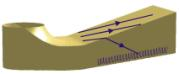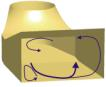Figure 2.7: Surface streamlines at the elbow and the first part of outlet diffuser.

Velocity and pressure measurements:

Section Ia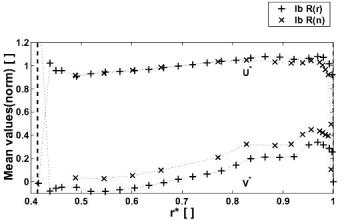Figure 2.8: The normalised mean velocity components at profile Ia(1).

Normalised axial and tangential velocity measurements at cross section Ia,
along profile 1 (-10°), for Case R(r). (See Figure 1.5).

Normalised axial and tangential velocity measurements at cross section Ia,
along profile 2 (180°), for Case R(r). (See Figure 1.5).

Normalised axial and tangential velocity measurements at cross section Ia,
along profile 1 (-10°), for Case R(n). (See Figure 1.5).

Normalised static pressure measurements at cross section Ia,
along profile 3 ( -80°), for Case R(n). (See Figure 1.5).

Section Ib

Normalised axial and tangential velocity measurements at cross section Ib,
along profile 1 (-80°), for Case R(r). (See Figure 1.5).

Normalised axial and tangential velocity measurements at cross section Ib,
along profile 1 (-80°), for Case R(n). (See Figure 1.5).

Section II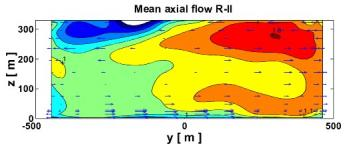Figure 2.9: The axial (contours) and horizontal (vectors) velocity components at c.s. II.

Normalised axial and tangential velocity and pressure measurements
at cross section II for Case R(n). (See Figure 1.4).

Section IVb

Normalised wall pressure measurements along at cross section IVb, for Case R(r).
(See Figure 1.4).

Centrelines

Normalised wall pressure measurements along the upper centre line, for Case R(n).
(See Figure 1.7).

Normalised wall pressure measurements along the lower centre line, for Case R(n).
(See Figure 1.7).